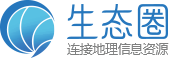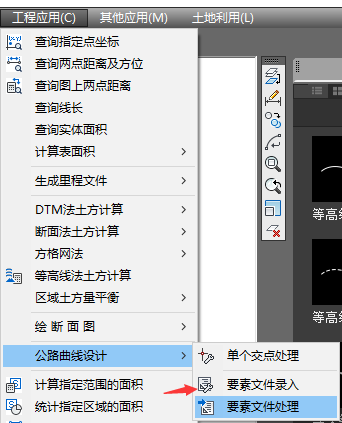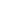# 南方cass绘图软件中的公路曲线设计文件是什么格式菜单截图

ANGLE，端点X坐标，端点Y坐标，起始里程，

JD交点序号，K里程公里数+里程不足公路数，X=交点的X坐标，Y=交点的Y坐标，A=偏角，R=圆曲线半径，T=切线长，Lh=缓和曲线长

ANGLE,X=0.000,Y=0.000,K0+0.000,A=0.0000,D=1624.030

JD1,A=91.0152,D=267.680,R=20.000,Lh=0.000

JD2,A=-90.0548,D=616.110,R=65.000,Lh=25.000

JD3,A=1.0804,D=374.420,R=5500.000,Lh=0.000

JD4,A=-1.1825,D=1011.640,R=5500.000,Lh=0.000

1、悬赏期为24小时，从发布时间起算；
2、悬赏期内提问者如设置评论为“精选评论”，视为悬赏结束，该精选评论人将获得本题全部悬赏积分；
3、悬赏期内如提问者未设置“精选评论”，则前5位评论者平分悬赏积分（如仅有一位评论，该评论者获得50%悬赏积分）；
4、悬赏积分会自动发放到生态圈账户中，请留意站内信通知。